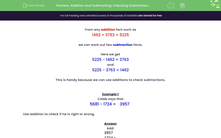# Check Subtraction Calculations by Using Addition

In this worksheet, students will use additions to check subtractions. Four-digit numbers are used.Key stage:  KS 2

Curriculum topic:   Number: Addition and Subtraction

Curriculum subtopic:   Estimate Calculations and Check Answers

Difficulty level:#### Worksheet Overview

From any addition fact such as

1,462 + 3,763 = 5,225

we can work out two subtraction facts.

Here we get

5,225 - 1,462 = 3,763

and

5,225 - 3,763 = 1,462

This is handy because we can use additions to check subtractions.

Example 1

Caleb says that

5,681 - 1,724 =
 3,957

Use addition to check if he is right or wrong.So 3,957 + 1,724 = 5,681

Caleb is right.Example 2

Dahlia says that

6,286 - 2,457 =
 4,829

Use addition to check if she is right or wrong.So 4,829 + 2,457 = 7,286 not 6,286

Dahlia is wrong.Let's have a go at some questions on this now.

### What is EdPlace?

We're your National Curriculum aligned online education content provider helping each child succeed in English, maths and science from year 1 to GCSE. With an EdPlace account you’ll be able to track and measure progress, helping each child achieve their best. We build confidence and attainment by personalising each child’s learning at a level that suits them.

Get started